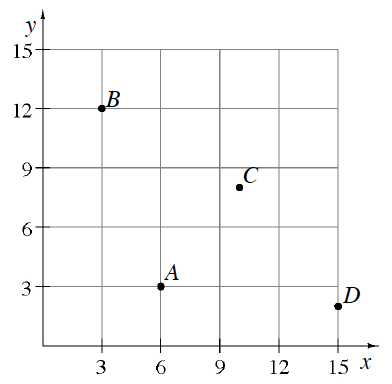### Home > CC1MN > Chapter 8 > Lesson 8.1.4 > Problem8-51

8-51.

Name the coordinates of each point shown in the graph at right using ordered pairs $(x, y)$.

$A=(6,3)$ and $B=(3,12)$.

Complete the ordered pairs for $C$ and $D$.